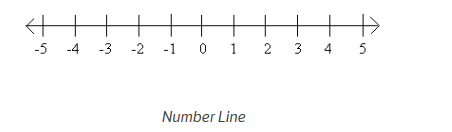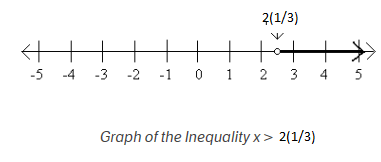# Introduction to Inequalities

## What is inequalities

• In mathematics, an inequality is a relation that holds between two values when they are different
• Solving linear inequalities is very similar to solving linear equations, except for one small but important detail: you flip the inequality sign whenever you multiply or divide the inequality by a negative

## Symbols used in inequalities

• The symbol < means less than. The symbol > means greater than.
• The symbol < with a bar underneath means less than or equal to. Usually this is written as $\leq$
• The symbol > with a bar underneath means greater than or equal to. Usually this is written as $\geq$
• The symbol $\neq$ means the quatities on left and right side are not equal

Examples
• a < b means a is less then b or b is greater a
• $a \leq b$ means a is less then or equal to b
• a > b means a is greater than b
• $a \geq b$ means a is greater or equal to b

## Things are which are safe to do in inequality which does not change in direction

• addition of same number on both sides
$a > b$
=> $a+c > b +c$
• Substraction of same number on both sides
$a > b$
=>$a-c > b-c$
• Multipication/Division by same positive number on both sides
$a > b$
if c is positive number then
$ac > bc$
or
$\frac {a}{c} > \frac {b}{c}$

## Things which changes the direction of the inequality

• swapping the left and right sides
• Multiplication/Division by negative number on both sides
• Dont multiple by variable whose values you dont know as you dont know the nature of the variable

## Concept Of Number line

• A number line is a horizontal line that has points which correspond to numbers. The points are spaced according to the value of the number they correspond to; in a number line containing only whole numbers or integers, the points are equally spaced.• It is very useful in solving problem related to inequalities and also representing it
Suppose x >2(1/ 3), this can represent this on number line like that## Linear Inequation in One Variable

A equation of the form
$ax+b > 0$
or
$ax+b \geq 0$
or
$ax+b< 0$
or
$ax+b \leq 0$
are called the linear equation in One Variable
Example:
• $x-2 < 0$
• $3x +10 > 0$
• $10x-17 \geq 0$

## Linear Inequation in Two Variable

A equation of the form
$ax+by > c$
or
$ax+by \geq c$
or
$ax+by< c$
or
$ax+by \leq c$
are called the linear equation in two Variable
Example:
• $x-2y < 0$
• $3x +10y > 0$
• $10x-17y \geq 0$